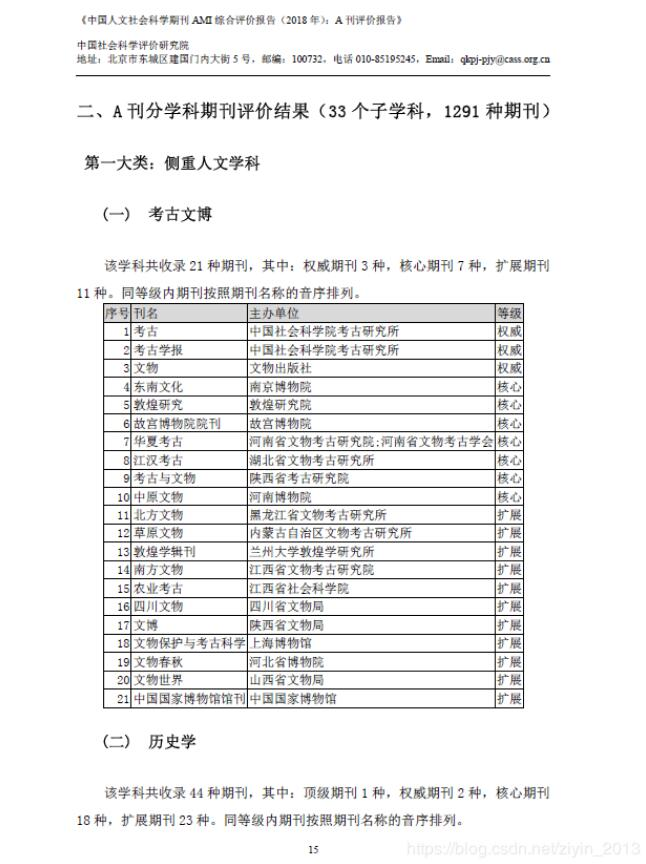﻿ python实现PDF中表格转化为Excel的方法_python_脚本之家
python# python实现PDF中表格转化为Excel的方法-pdfminer:擅长文字的解析，把表格解析成普通的文本，没有格式；
-pdf2html:把pdf解析成html，但html的标签并没有规律，解析一个表格还可以，多个表格的话不太好提取；
-tabula:对于简单的表格，即单元格中没有换行的，表头表尾形式不复杂的，使用比较方便。但是单脑需要Java环境；
-pdfplumber:是一个可以处理pdf格式信息的库。可以查找关于每个文本字符、矩阵、和行的详细信息，也可以对表格进行提取并进行可视化调试。

.pages是一个包含页面信息的列表。

-pdfplumber.page的类中包含的主要的属性：

.page_number 页码。
.width 页面宽度。
.height 页面高度。
.objects/.chars/.lines/.rects 这些属性中每一个都是一个列表，每个列表都包含一个字典，每个字典用于说明页面中的对象信息， 包括直线，字符， 方格等位置信息。

-一些常用的方法：

.extract_text() 用来提页面中的文本，将页面的所有字符对象整理为的那个字符串。
.extract_words() 返回的是所有的单词及其相关信息。
.extract_tables() 提取页面的表格。
.to_image() 用于可视化调试时，返回PageImage类的一个实例。

```import pdfplumber
import pandas as pd

path = 'test.pdf'
pdf = pdfplumber.open(path)
i=1
#writer=pd.ExcelWriter('output.xlsx')
df=pd.DataFrame(columns=['序号','刊名','主办单位','等级'])
sheetname=['考古文博','历史学','马克思主义理论','民族学与文化学','文学-外国文学','文学-中国文学','艺术学','语言学','哲学','宗教学','法学'
,'管理学','环境科学','教育学','经济学-财政科学','经济学-工业经济','经济学-金融','经济学-经济管理','经济学-经济综合','经济学-贸易经济'
,'经济学-农业经济','经济学-世界经济','人文地理学','社会学','体育学','统计学','图书馆情报与档案学','心理学','新闻学与传播学'
,'政治学-国际政治','政治学-中国政治','综合-高校综合性学报','综合-综合性人文社科期刊']

##由于存在一个表格跨页的情况，先将所有表格存放在一个DataFrame中，再根据序号拆分。

for page in pdf.pages[17:59]:
print (page)
# 获取当前页面的全部文本信息，包括表格中的文字
# print(page.extract_text())
for table in page.extract_tables():
#print(table)
df=df.append(pd.DataFrame(table[1:],columns=table),ignore_index=True)
print (df)

writer=pd.ExcelWriter('output3.xlsx')
new_df=pd.DataFrame()
j=1
index=[]
#记录序号==1的行索引，用于后面的表格拆分
for i in range(len(df)):
if df.ix[i,0]=='1':
index.append(i)
print ("################")
index.append(len(df))
#print (index)

#按行索引将内容切片并逐个添加到表中
for t in range(len(index)-1):
new_df=df.ix[index[t]:index[t+1]-1,:]
#print (new_df)
new_df.to_excel(writer,sheet_name=sheetname[t],encoding='gb2312',index=None)
writer.save()
pdf.close()
print('finished') ```